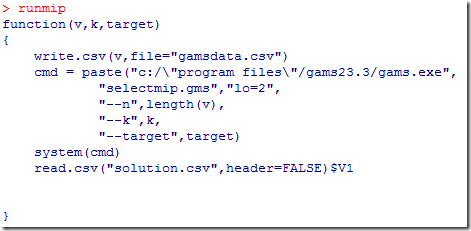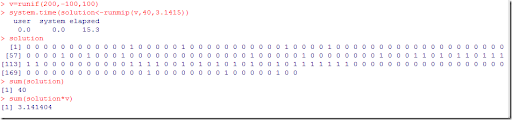## Sunday, January 31, 2010

### Run GAMS from R

This is a simplistic way to run GAMS from R:The GAMS model is adapted from here. It looks like:

\$ontext

Linear version

\$offtext

*
* data
*
set i /1*%n%/;
scalars
k
/%k%/
target
/%target%/
;

table pt(i,*)
\$ondelim
\$include gamsdata.csv
\$offdelim
;

parameter p(i);
p(i) = pt(i,
"x");

*
* model
*
binary variable x(i);
variable z,s,d1,d2;
positive variable d1,d2;

equation e1,e2,e3,obj;

e1.. s =e=
sum(i, p(i)*x(i));
e2..
sum(i,x(i)) =e= k;
e3.. d1-d2 =e= s-target;
obj.. z =e= d1+d2;

model m /all/;
option optcr=0, mip=cplex;
solve m minimizing z using mip;

*
* do some reporting
*

option x:1;
display p,x.l;

scalar value,sqdeviation,num;
value =
sum(i, p(i)*x.l(i));
sqdeviation = sqr(value  - target);
num =
sum(i, x.l(i));
display target,value,sqdeviation,num;

*
* write solution
*
file sol /solution.csv/;
loop(i,

put sol,x.l(i)/
);

Here is how to use it:#### 1 comment:

1.Do you have an example for running R from GAMS? I would appreciate your kind help if you provied a demo file.

e-mail ID: shyam.kumar.basnet@slu.se

Thanks,
Shyam